﻿ Math 12 Honours
 FAIL (the browser should render some flash content, not this).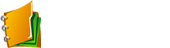Math 12 Honours Ch 1

Basic Functions

In this chapter, students will learn:

• Translate a function horizontally and vertically
• Reflection a function over both axis
• Expand and compress a function horizontally and vertically
• Graph the inverse of a function

Click for Homework

PPT

•  1.1
•  1.2
•  1.3
•  1.4
•  1.5
•  1.6
•  1.5R
•  1.6RAt BCMath.ca we offer hundreds of free online math lessons for students and teachers. Help support our site by clicking on the button above to donate through Paypal.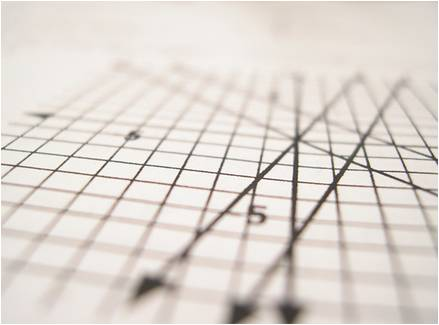Math 12 Honours Ch 2

Transformations and Functions
Tool Kit

In this chapter, students will learn:

• Apply transformations to Trigonometric functions
• Solve advanced trigonometric equations using identities and transformations
• Explore basic applications of trigonometric equations

Click for Homework

PPT

•  2.1
•  2.2
•  2.3
•  2.4
•  2.5
•  2.6
•  Ch2R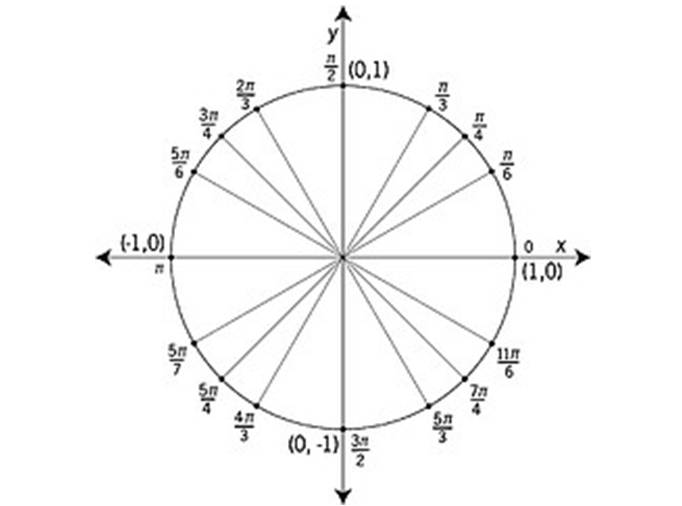Math 12 Honours Ch 3
•

Polynomials and Rational Functions
In this chapter, students will learn:

• Apply transformations to Trigonometric functions
• Solve advanced trigonometric equations using identities and transformations
• Explore basic applications of trigonometric equations

Click for Homework

PPT

•  3.1
•  3.2
•  3.3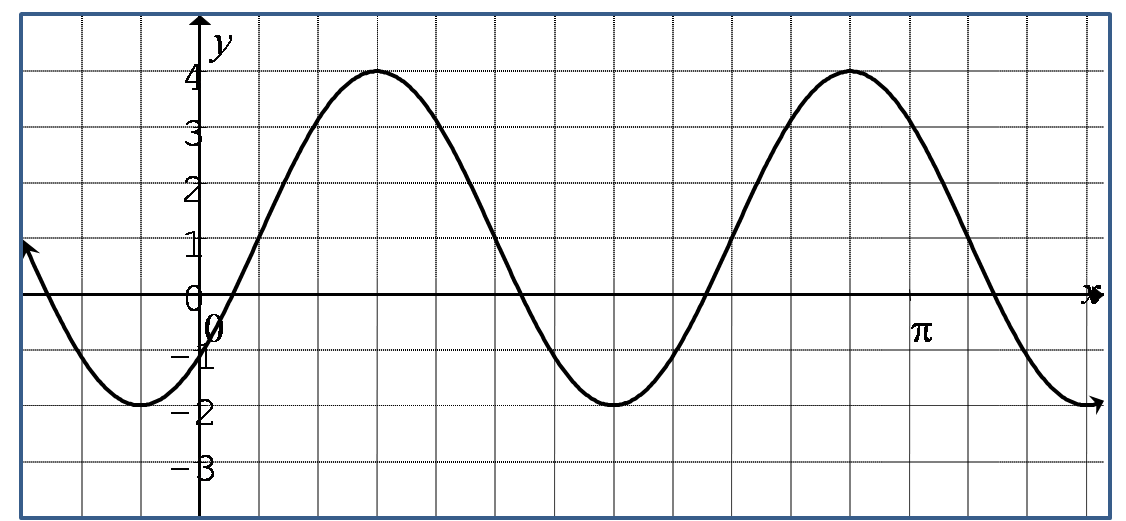Math 12 Honours Ch 4

Transformations with Trigonomery
In this chapter, students will learn:

• to solve basic equations involving exponents and logarithms
• to graph logarithmic and exponential functions with transformations
• basic applications of logarithm and exponents
• Solve advanced equations with Logarithm identities

PPT

•  4a
•  4.1
•  4.2
•  4.3
•  4.4
•  4.5
•  4.6
•  4.7Math 12 Honours Ch 5

Exponents and Logarithms

In this chapter, students will learn:

• to prove basic geometry concents with coordinate geometry
• to graph Circles, Ellipses, and Hyperbolas with transformations
• to calculate the shortest distance from any point to a line

PPT

•  5.1
•  5.2
•  5.3
•  5.4
•  5.5
•  5.6
•  5.7
•  5.8
•  Ch5R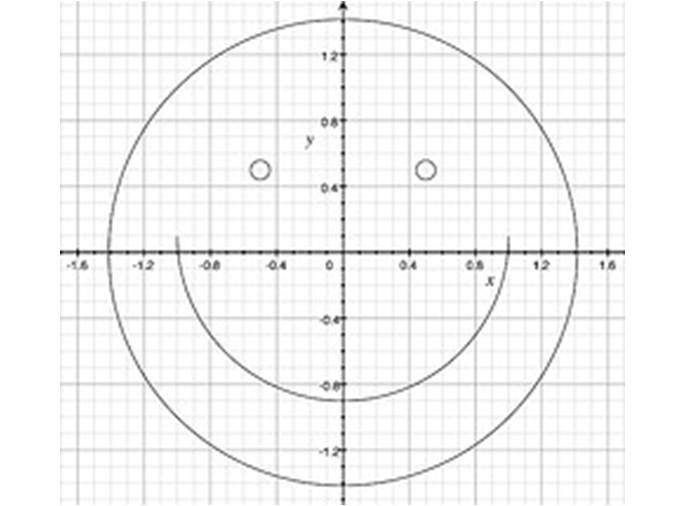Math 12 Honours Ch 6

Relations and CONICS

In this chapter, students will learn:

• Basic properties of Matrices
• Applications of Matrices in Linear Algebra

PPT
•  6.1
•  6.2
•  6.3
•  6.4
•  6.5
•  6.6Math 12 Honours Ch 7

•  Section 7.1: What are Limits
•  Section 7.1: What are Limits

Limits

In this chapter, students will learn:

• how to calculate the limit of a function
• Applications of Limits

PPT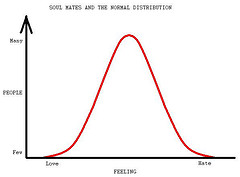Math 12 Honours Ch 8

•  Section 8.1: Functions & Transformations
•  Section 8.2: Logarithms & Exponentials
•  Section 8.3: Trigonometric Functions
•  Section 8.4: Graphing Trigonometric Functions

Statistics

•  Section 8.5: Trigonometric Identities
•  Section 8.6: Counting Principles
•  Section 8.7: Probability
•  Section 8.8: Statistics
•  Section 8.9: Circles, Ellipses, and Conics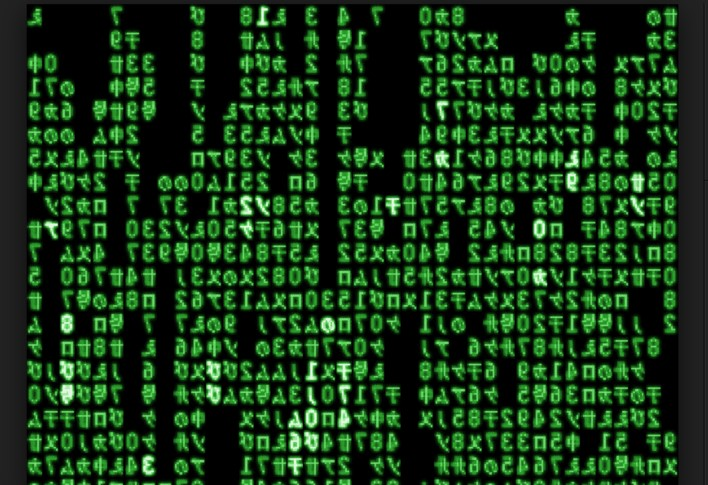Math 12 Honours Ch 9

•  Section 9.1: Functions & Transformations
•  Section 9.2: Logarithms & Exponentials
•  Section 9.3: Trigonometric Functions
•  Section 9.4: Graphing Trigonometric Functions

Linear Algebra and Matrices

•  Section 9.5: Trigonometric Identities
•  Section 9.6: Counting Principles
•  Section 9.7: Probability
•  Section 9.8: Statistics
•  Section 9.9: Circles, Ellipses, and Conics